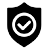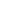#### WhatsApp Quick Chat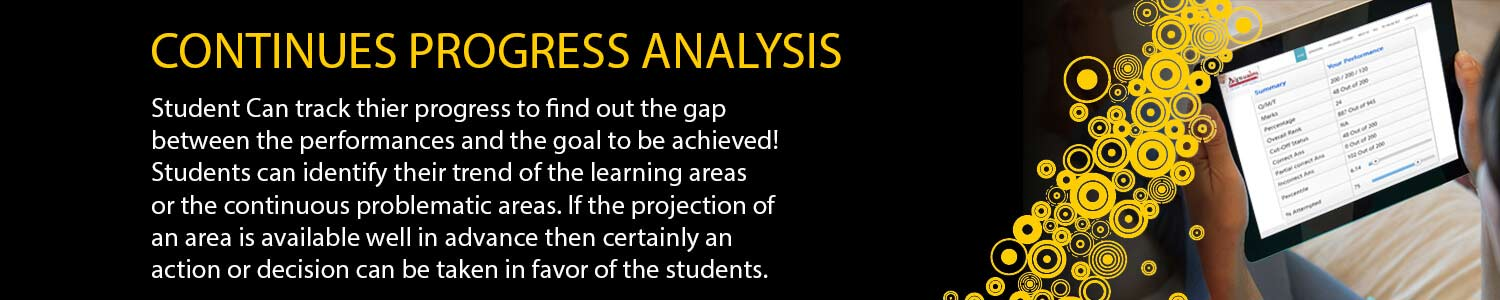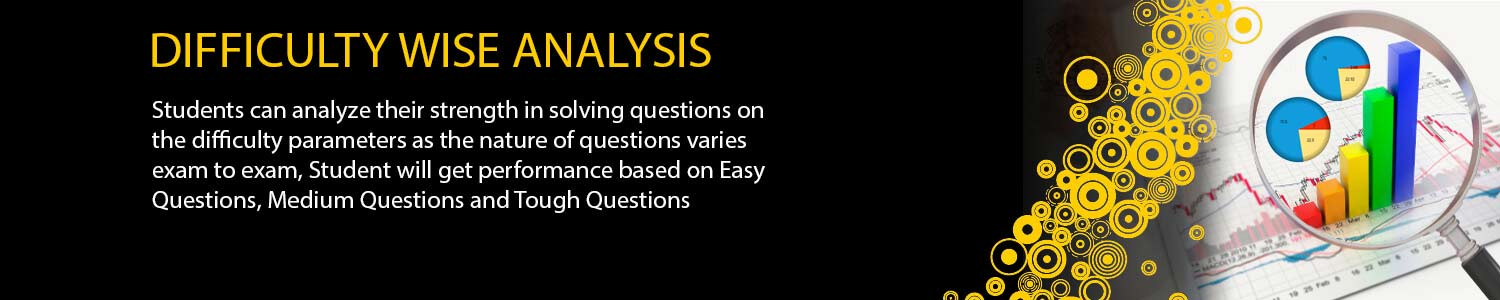## CSIR NET / JRF

#### Offers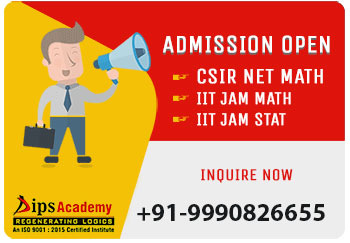##### News & Events# NET JRF Online Test Series

Today era is highly influenced by Information Technology and thus most crucial exams conducted online and since these exams are necessary for the scholars student as they achieve success by clearing them only For example CSIR, Gate and JAM. Most number of students of DIPS Academy got selected in these exams because our students experience the real exams temperament from the online test platform which is used by DIPS Academy.

Our Online test platform is best in India and more or less same used by exams conducting body. All the students of DIPS Academy are provided by the unique User ID and Password. Students not only able to give the test by using these ID’s but also get a comprehensive analysis.

Students from pan India can get the benefits of our Online Test. Our test Package is differentiated according to the needs of students and they can choose them as per their requirement which is not only helping them in preparation of exams but also they can check their all India Ranking. Our Online Test are merged with the classroom test so that students can compete themselves from our regular students and most sincere aspirants of the exams.

#### Main features of our Test Series

High standard of question

Questions are chosen as per the weightage of topic in the real exam

Questions are available on each and every minute concept.

Practicing of test paper helps you in understanding the whole concept.

Full Length, Concept/Module wise tests available

Full analysis of your performance on the test

Get Answer key and solution post test

Papers are designed as the real pattern.

You can attempt the paper as per your own convenience#### NET All India Ranker

(Online Test Series)

12 Test ( 8 Module Wise + 4 Full Length)#### NET Real Time

(Online Test Series)

8 Full Length Test ( 4 New + 4 Old )#### NET Anytime

(Test Series CSIR NET)

24 Test (12 New + 12 Old)

### TEST SERIES SCHEDULE ( CSIR-NET/JRF MAY-2023 ONLINE)

Date TEST TYPE Modules Syllabus
MWT-01 Real Analysis I 1-May-23 Elementary set theory, finite, countable and uncountable sets, Real number system as a complete ordered field, Archimedean property, supremum, infimum. Sequences and series, convergence, limsup, liminf. Bolzano Weierstrass theorem, Heine Borel theorem. Continuity,monotonic functions, types of discontinuity, uniform continuity, Metric spaces, compactness, connectedness.
MWT-02 ODE & IE 4-May-23 Existence and uniqueness of solutions of initial value problems for first order ordinary differential equations, singular solutions of first order ODEs, system of first order ODEs. General theory of homogenous and non-homogeneous linear ODEs, variation of parameters, Sturm-Liouville boundary value problem. Linear integral equation of the first and second kind of Fredholm and Volterra type, Solutions with separable kernels. Characteristic numbers and eigenfunctions, resolvent kernel.
MWT-03 Real Analysis II & Functional Analysis 7-May-23 differentiability, mean value theorem. Sequences and series of functions, uniform convergence. Riemann sums and Riemann integral, Improper Integrals. functions of bounded variation, Lebesgue measure, Lebesgue integral. Functions of several variables, directional derivative, partial derivative, derivative as a linear transformation, inverse and implicit function theorems. Metric spaces, Topology, compactness, connectedness.
MWT-04 Linear Algebra -I 11-May-23 Vector spaces, subspaces, linear dependence, basis, dimension, algebra of linear transformations, matrix representation of linear transformation, Algebra of matrices, rank and determinant of matrices, system of linear equations.
MWT-05 PDE & COV 14-May-23 Variation of a functional, Euler-Lagrange equation, Necessary and sufficient conditions for extrema. Variational methods for boundary value problems in ordinary and partial differential equations. Green’s function. Lagrange and Charpit methods for solving first order PDEs, Cauchy problem for first order PDEs. Classification of second order PDEs, General solution of higher order PDEs with constant coefficients, Method of separation of variables for Laplace, Heat and Wave equations.
MWT-06 Linear Algebra-II 17-May-23 Eigenvalues and eigenvectors, Cayley-Hamilton theorem. Change of basis, canonical forms, diagonal forms, triangular forms, Jordan forms. Inner product spaces, orthonormal basis. Quadratic forms, reduction and classification of quadratic forms
MWT-07 Group Theory & Ring Theory 20-May-23 Permutations, combinations, Fundamental theorem of arithmetic, divisibility in Z, congruences, Chinese Remainder Theorem, Euler’s Ø- function, primitive roots. Groups, subgroups, normal subgroups, quotient groups, homomorphisms, cyclic groups, permutation groups, Cayley’s theorem, class equations, Sylow theorems.Rings, ideals, prime and maximal ideals, quotient rings, unique factorization domain, principal ideal domain, Euclidean domain. Polynomial rings and irreducibility criteria. Fields, finite fields, field extensions,
MWT-08 Complex Analysis 23-May-23 Algebra of complex numbers, the complex plane, polynomials, power series, transcendental functions such as exponential, trigonometric and hyperbolic functions. Analytic functions, Cauchy-Riemann equations. Contour integral, Cauchy’s theorem, Cauchy’s integral formula, Liouville’s theorem, Maximum modulus principle, Schwarz lemma, Open mapping theorem. Taylor series, Laurent series, calculus of residues. Conformal mappings, Mobius transformations.
FLT-01 Full Length Test 26-May-23 As per Exam Pattern
FLT-02 Full Length Test 29-May-23 As per Exam Pattern
FLT-03 Full Length Test 1-Jun-23 As per Exam Pattern
FLT-04 Full Length Test 4-Jun-23 As per Exam Pattern

## Offers## News & Events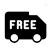##### Free Delivery